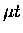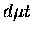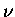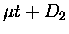Next: Formulation of the formation Up: Resonant molecular formation Previous: Resonant molecular formation

## The standard Vesman model

As mentioned earlier, resonant molecular formation refers to the process:(38)

where the kinetic energy ofand the energy released upon formation ofis absorbed in the rotational (K) and vibrational () excitation of the molecular complex, a hydrogen like molecule withplaying the role of one of the nuclei. The process is resonant in nature because of the discrete spectra in the final states, corresponding mainly to the ro-vibrational levels (, and the collision energy has to match the resonant condition. The resonance condition can be written, when considering purely two body collisions (i.e., neglecting three-body or phonon effects):(39)

Figure 2.3 gives a schematic energy level diagram for the resonantformation process. Plotted on the left is the potential curve ofshowing the shallow'' (in the muonic scale) bound state, while on the right the molecular complex energy levels are plotted. Note that due to the reduced mass difference, the energy levels of D2 and [] are different (by 33.7 meV for the ground states according to Faifman et al. ). In fact it is this difference in reduced mass which introduces the dependence of theformation rates on the target molecular species, i.e.,versusor.Under ordinary target conditions, onlyis populated. On the other hand, the initial rotational population depends on the target preparation procedure as well as on temperature. At 3 K, an equilibrated target would have nearly 100% Ki=0 population (ortho deuterium). Our targets, however, were prepared by rapidly freezing the statistically populated deuterium (67% Ki = 0 and 33% Kf = 1) from a hot palladium filter, and because the rotational relaxation is very slow in the absence of a catalyst, we expect this population will last for the entire measurement time. The initial ortho-para population is relevant, because angular momentum conservation requires:(40)

where L is the relative angular momentum in the reaction (2.24). Since therotational angular momentum J is 1, we havefor low energy collisions with L=0 . However, at epithermal energies L>0 becomes important, allowing various Kf states. This is one of the reasons why we expect such a high rate at epithermal energies.Next: Formulation of the formation Up: Resonant molecular formation Previous: Resonant molecular formation